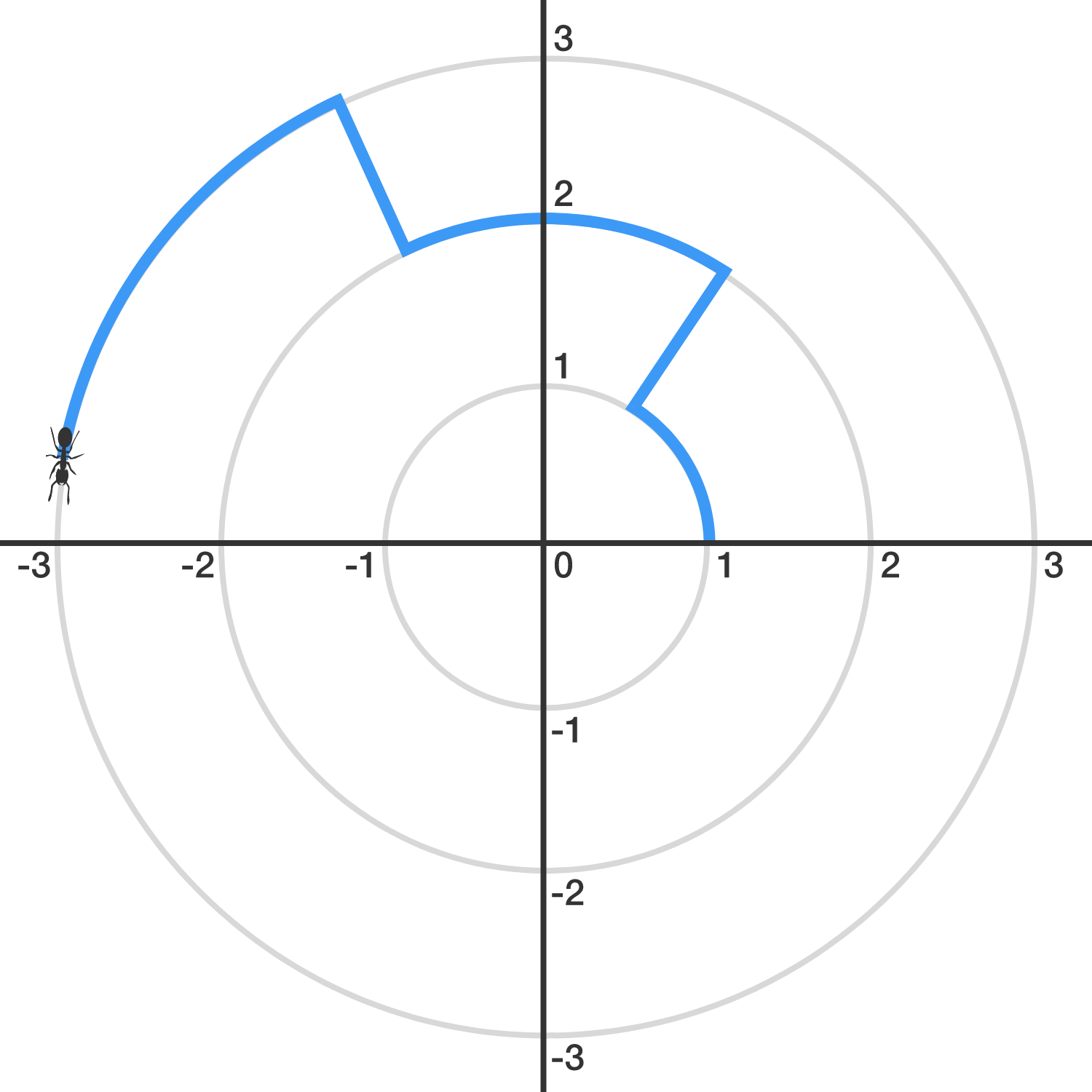Geometry

# Trigonometric Functions: Level 3 Challenges

Find the value of $-\sin^2{1^\circ}+\sin^2{2^\circ }-\sin^2{3^\circ }+\ldots+\sin^2{88^\circ}-\sin^2{89^\circ}+\sin^2{90^\circ}$

$\left [ \log_{10} \left ( \tan \left ( 1^\circ \right ) \right ) \right ] \times \left [ \log_{10} \left ( \tan \left ( 2^\circ \right ) \right ) \right ] \times \left [ \log_{10} \left ( \tan \left ( 3^\circ \right ) \right ) \right ] \times \cdot \cdot \cdot \\ \times \left [ \log_{10} \left ( \tan \left ( 88^\circ \right ) \right ) \right ] \times \left [ \log_{10} \left ( \tan \left ( 89^\circ \right ) \right ) \right ] = \ ?$An ant finds itself trapped in the $$xy$$-plane, and its initial position is $$(1,0).$$

Let $$S_k$$ denote the circle with radius $$k$$ centered around the origin. Starting from $$(1,0)$$, the ant walks 1 unit counter-clockwise on $$S_{1}.$$ Then, it walks directly (radially outward) to $$S_2,$$ on which it will walk 2 units counter-clockwise. Then, it will walk directly to $$S_3$$ and walk 3 units counter-clockwise, and so, with the ant walking $$k$$ units on $$S_k.$$ (See the image above.)

When the ant crosses the positive $$x$$-axis for the first time since it left $$(1,0)$$, it is on $$S_{n}$$. What is $$n$$?

Find the value of

$\frac{\cos^4 75^{\circ}+\sin^4 75^{\circ}+3\sin^2 75^{\circ}\cos^2 75^{\circ}}{\cos^6 75^{\circ}+\sin^6 75^{\circ}+4\sin^2 75^{\circ}\cos^2 75^{\circ}}.$

If the domain of $f(x)=\tan^{-1} \left( \dfrac{x^2+1}{x^2+\sqrt{3}}\right)$ is the real numbers, find its range.

×

Problem Loading...

Note Loading...

Set Loading...